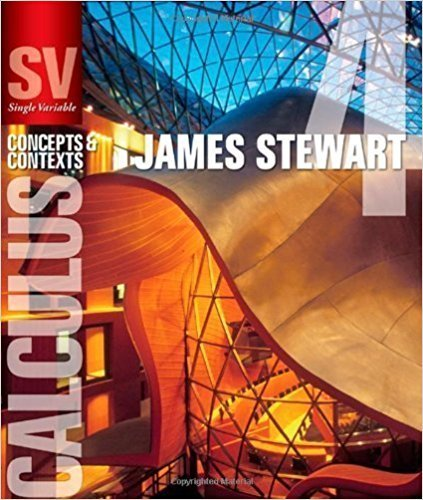×
×

# Solutions for Chapter 2.5: LIMITS INVOLVING INFINITY## Full solutions for Single Variable Calculus: Concepts and Contexts (Stewart's Calculus Series) | 4th Edition

ISBN: 9780495559726Solutions for Chapter 2.5: LIMITS INVOLVING INFINITY

Solutions for Chapter 2.5
4 5 0 300 Reviews
27
1
##### ISBN: 9780495559726

Chapter 2.5: LIMITS INVOLVING INFINITY includes 58 full step-by-step solutions. This textbook survival guide was created for the textbook: Single Variable Calculus: Concepts and Contexts (Stewart's Calculus Series), edition: 4. This expansive textbook survival guide covers the following chapters and their solutions. Single Variable Calculus: Concepts and Contexts (Stewart's Calculus Series) was written by and is associated to the ISBN: 9780495559726. Since 58 problems in chapter 2.5: LIMITS INVOLVING INFINITY have been answered, more than 22424 students have viewed full step-by-step solutions from this chapter.

Key Calculus Terms and definitions covered in this textbook
• Arctangent function

See Inverse tangent function.

• Complements or complementary angles

Two angles of positive measure whose sum is 90°

• Cosecant

The function y = csc x

• Degree

Unit of measurement (represented by the symbol ) for angles or arcs, equal to 1/360 of a complete revolution

• Equal matrices

Matrices that have the same order and equal corresponding elements.

• Equilibrium point

A point where the supply curve and demand curve intersect. The corresponding price is the equilibrium price.

• Exponential growth function

Growth modeled by ƒ(x) = a ? b a > 0, b > 1 .

• Increasing on an interval

A function ƒ is increasing on an interval I if, for any two points in I, a positive change in x results in a positive change in.

• Inductive step

See Mathematical induction.

• Infinite limit

A special case of a limit that does not exist.

• Integrable over [a, b] Lba

ƒ1x2 dx exists.

• Interval

Connected subset of the real number line with at least two points, p. 4.

• Local maximum

A value ƒ(c) is a local maximum of ƒ if there is an open interval I containing c such that ƒ(x) < ƒ(c) for all values of x in I

• Main diagonal

The diagonal from the top left to the bottom right of a square matrix

• Mapping

A function viewed as a mapping of the elements of the domain onto the elements of the range

• Sequence of partial sums

The sequence {Sn} , where Sn is the nth partial sum of the series, that is, the sum of the first n terms of the series.

• Standard deviation

A measure of how a data set is spread

• Subtraction

a - b = a + (-b)

• Triangular number

A number that is a sum of the arithmetic series 1 + 2 + 3 + ... + n for some natural number n.

• Yscl

The scale of the tick marks on the y-axis in a viewing window.

×